Evaluate temperature gradient of a thermal solution at arbitrary spatial locations

Description

example

[gradTx,gradTy] = evaluateTemperatureGradient(thermalresults,xq,yq) returns the interpolated values of temperature gradients of the thermal model solution thermalresults at the 2-D points specified in xq and yq. This syntax is valid for both the steady-state and transient thermal models.

example

example

[___] = evaluateTemperatureGradient(thermalresults,querypoints) returns the interpolated values of the temperature gradients at the points specified in querypoints. This syntax is valid for both the steady-state and transient thermal models.

example

[___] = evaluateTemperatureGradient(___,iT) returns the interpolated values of the temperature gradients for the time-dependent equation at times iT. Specify iT after the input arguments in any of the previous syntaxes.

The first dimension of gradTx, gradTy, and, in 3-D case, gradTz corresponds to query points. The second dimension corresponds to time-steps iT.

Examples

collapse all

For a 2-D steady-state thermal model, evaluate temperature gradients at the nodal locations and at the points specified by x and y coordinates.

Create a thermal model for steady-state analysis.

thermalmodel = createpde('thermal');

Create the geometry and include it in the model.

R1 = [3,4,-1,1,1,-1,1,1,-1,-1]';
g = decsg(R1,'R1',('R1')');
geometryFromEdges(thermalmodel,g);
pdegplot(thermalmodel,'EdgeLabels','on')
xlim([-1.5 1.5])
axis equalAssuming that this geometry represents an iron plate, the thermal conductivity is $79.5\phantom{\rule{0.16666666666666666em}{0ex}}W/\left(mK\right)$.

thermalProperties(thermalmodel,'ThermalConductivity',79.5,'Face',1);

Apply a constant temperature of 300 K to the bottom of the plate (edge 3). Also, assume that the top of the plate (edge 1) is insulated, and apply convection on the two sides of the plate (edges 2 and 4).

thermalBC(thermalmodel,'Edge',3,'Temperature',300);
thermalBC(thermalmodel,'Edge',1,'HeatFlux',0);
thermalBC(thermalmodel,'Edge',[2 4], ...
'ConvectionCoefficient',25, ...
'AmbientTemperature',50);

Mesh the geometry and solve the problem.

generateMesh(thermalmodel);
results = solve(thermalmodel)
results =

Temperature: [1541x1 double]
Mesh: [1x1 FEMesh]

The solver finds the temperatures and temperature gradients at the nodal locations. To access these values, use results.Temperature, results.XGradients, and so on. For example, plot the temperature gradients at nodal locations.

figure;
pdeplot(thermalmodel, ...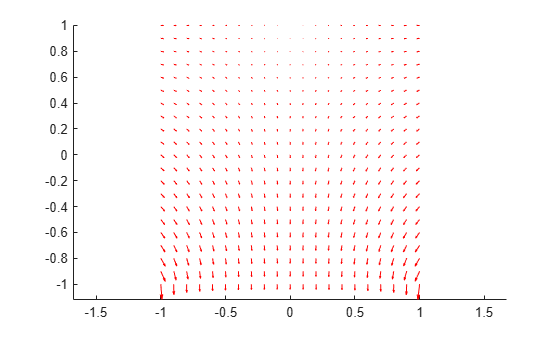Create a grid specified by x and y coordinates, and evaluate temperature gradients to the grid.

v = linspace(-0.5,0.5,11);
[X,Y] = meshgrid(v);

figure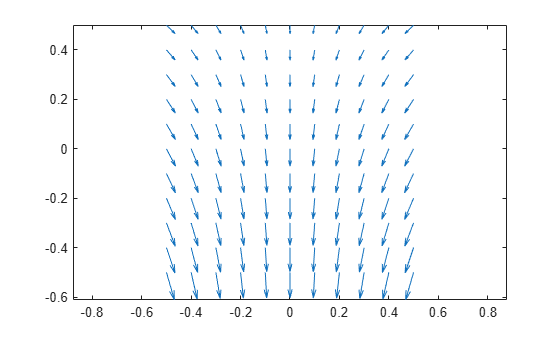Alternatively, you can specify the grid by using a matrix of query points.

querypoints = [X(:) Y(:)]';

figureFor a 3-D steady-state thermal model, evaluate temperature gradients at the nodal locations and at the points specified by x, y, and z coordinates.

Create a thermal model for steady-state analysis.

thermalmodel = createpde('thermal');

Create the following 3-D geometry and include it in the model.

importGeometry(thermalmodel,'Block.stl');
pdegplot(thermalmodel,'FaceLabels','on','FaceAlpha',0.5)
title('Copper block, cm')
axis equal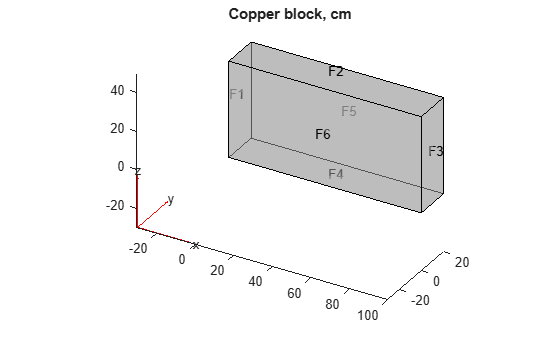Assuming that this is a copper block, the thermal conductivity of the block is approximately $4\phantom{\rule{0.16666666666666666em}{0ex}}W/\left(cmK\right)$.

thermalProperties(thermalmodel,'ThermalConductivity',4);

Apply a constant temperature of 373 K to the left side of the block (edge 1) and a constant temperature of 573 K to the right side of the block.

thermalBC(thermalmodel,'Face',1,'Temperature',373);
thermalBC(thermalmodel,'Face',3,'Temperature',573);

Apply a heat flux boundary condition to the bottom of the block.

thermalBC(thermalmodel,'Face',4,'HeatFlux',-20);

Mesh the geometry and solve the problem.

generateMesh(thermalmodel);
thermalresults = solve(thermalmodel)
thermalresults =

Temperature: [12691x1 double]
Mesh: [1x1 FEMesh]

The solver finds the values of temperatures and temperature gradients at the nodal locations. To access these values, use results.Temperature, results.XGradients, and so on.

Create a grid specified by x, y, and z coordinates, and evaluate temperature gradients to the grid.

[X,Y,Z] = meshgrid(1:26:100,1:6:20,1:11:50);

figure
axis equal
xlabel('x')
ylabel('y')
zlabel('z')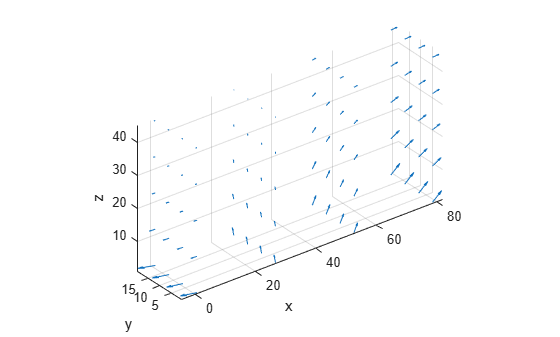Alternatively, you can specify the grid by using a matrix of query points.

querypoints = [X(:) Y(:) Z(:)]';

figure
axis equal
xlabel('x')
ylabel('y')
zlabel('z')Solve a 2-D transient heat transfer problem on a square domain and compute temperature gradients at the convective boundary.

Create a transient thermal model for this problem.

thermalmodel = createpde('thermal','transient');

Create the geometry and include it in the model.

g = @squareg;
geometryFromEdges(thermalmodel,g);
pdegplot(thermalmodel,'EdgeLabels','on')
xlim([-1.2 1.2])
ylim([-1.2 1.2])
axis equalAssign the following thermal properties: thermal conductivity is $100\phantom{\rule{0.16666666666666666em}{0ex}}W/\left({m}^{\circ }C\right)$, mass density is $7800\phantom{\rule{0.16666666666666666em}{0ex}}kg/{m}^{3}$, and specific heat is $500\phantom{\rule{0.16666666666666666em}{0ex}}J/\left(k{g}^{\circ }C\right)$.

thermalProperties(thermalmodel,'ThermalConductivity',100, ...
'MassDensity',7800, ...
'SpecificHeat',500);

Apply insulated boundary conditions on three edges and the free convection boundary condition on the right edge.

thermalBC(thermalmodel,'Edge',[1 3 4],'HeatFlux',0);
thermalBC(thermalmodel,'Edge',2, ...
'ConvectionCoefficient',5000, ...
'AmbientTemperature',25);

Set the initial conditions: uniform room temperature across domain and higher temperature on the left edge.

thermalIC(thermalmodel,25);
thermalIC(thermalmodel,100,'Edge',4);

Generate a mesh and solve the problem using 0:1000:200000 as a vector of times.

generateMesh(thermalmodel);
tlist = 0:1000:200000;
thermalresults = solve(thermalmodel,tlist);

Define a line at convection boundary and compute temperature gradients across that line.

X = -1:0.1:1;
Y = ones(size(X));

X,Y,1:length(tlist));

Plot the interpolated gradient component gradTx along the x axis for the following values from the time interval tlist.

figure
t = [51:50:201];
for i = t
strcat('t=',num2str(tlist(i))));
hold on
end
legend(p(t))
xlabel('x')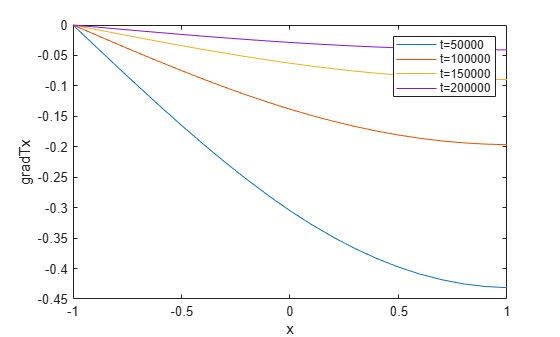Input Arguments

collapse all

Solution of a thermal problem, specified as a SteadyStateThermalResults object or a TransientThermalResults object. Create thermalresults using the solve function.

Example: thermalresults = solve(thermalmodel)

x-coordinate query points, specified as a real array. evaluateTemperatureGradient evaluates temperature gradient at the 2-D coordinate points [xq(i) yq(i)] or at the 3-D coordinate points [xq(i) yq(i) zq(i)]. So xq, yq, and (if present) zq must have the same number of entries.

evaluateTemperatureGradient converts query points to column vectors xq(:), yq(:), and (if present) zq(:). It returns the temperature gradient in a form of a column vector of the same size. To ensure that the dimensions of the returned solution is consistent with the dimensions of the original query points, use reshape. For example, use gradTx = reshape(gradTx,size(xq)).

Data Types: double

y-coordinate query points, specified as a real array. evaluateTemperatureGradient evaluates the temperature gradient at the 2-D coordinate points [xq(i) yq(i)] or at the 3-D coordinate points [xq(i) yq(i) zq(i)]. So xq, yq, and (if present) zq must have the same number of entries.

evaluateTemperatureGradient converts query points to column vectors xq(:), yq(:), and (if present) zq(:). It returns the temperature gradient in a form of a column vector of the same size. To ensure that the dimensions of the returned solution is consistent with the dimensions of the original query points, use reshape. For example, use gradTy = reshape(gradTy,size(yq)).

Data Types: double

z-coordinate query points, specified as a real array. evaluateTemperatureGradient evaluates the temperature gradient at the 3-D coordinate points [xq(i) yq(i) zq(i)]. So xq, yq, and zq must have the same number of entries.

evaluateTemperatureGradient converts query points to column vectors xq(:), yq(:), and (if present) zq(:). It returns the temperature gradient in a form of a column vector of the same size. To ensure that the dimensions of the returned solution is consistent with the dimensions of the original query points, use reshape. For example, use gradTz = reshape(gradTz,size(zq)).

Data Types: double

Query points, specified as a real matrix with either two rows for 2-D geometry, or three rows for 3-D geometry. evaluateTemperatureGradient evaluates the temperature gradient at the coordinate points querypoints(:,i), so each column of querypoints contains exactly one 2-D or 3-D query point.

Example: For 2-D geometry, querypoints = [0.5 0.5 0.75 0.75; 1 2 0 0.5]

Data Types: double

Time indices, specified as a vector of positive integers. Each entry in iT specifies a time index.

Example: iT = 1:5:21 specifies every fifth time-step up to 21.

Data Types: double

Output Arguments

collapse all

x-component of the temperature gradient, returned as a matrix. For query points that are outside the geometry, gradTx = NaN.

y-component of the temperature gradient, returned as a matrix. For query points that are outside the geometry, gradTy = NaN.

z-component of the temperature gradient, returned as a matrix. For query points that are outside the geometry, gradTz = NaN.